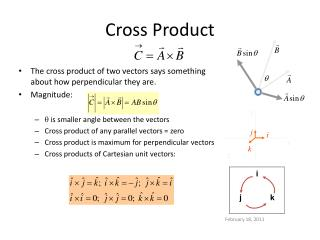DownloadDownload PresentationCross Product

# Cross Product

Download Presentation## Cross Product

- - - - - - - - - - - - - - - - - - - - - - - - - - - E N D - - - - - - - - - - - - - - - - - - - - - - - - - - -
##### Presentation Transcript

1. y q j i x i k z j k Cross Product • The cross product of two vectors says something about how perpendicular they are. • Magnitude: •  is smaller angle between the vectors • Cross product of any parallel vectors = zero • Cross product is maximum for perpendicular vectors • Cross products of Cartesian unit vectors:

2. Cross Product • Direction: C perpendicular to both A and B (right-hand rule) • Place A and B tail to tail • Right hand, not left hand • Four fingers are pointed along the first vector A • “sweep” from first vector A into second vector B through the smaller angle between them • Your outstretched thumb points the direction of C • First practice

3. More about Cross Product • The quantity ABsin is the area of the parallelogram formed by A and B • The direction of C is perpendicular to the plane formed by A and B • Cross product is not commutative • The distributive law • The derivative of cross product obeys the chain rule • Calculate cross product

4. Derivation • How do we show that ? • Start with • Then • But • So

5. Torque as a Cross Product • The torque is the cross product of a force vector with the position vector to its point of application • The torque vector is perpendicular to the plane formed by the position vector and the force vector (e.g., imagine drawing them tail-to-tail) • Right Hand Rule: curl fingers from r to F, thumb points along torque. Superposition: • Can have multiple forces applied at multiple points. • Direction of tnetis angular acceleration axis